# GSEB Solutions Class 9 Science Chapter 11 Work and Energy

Gujarat Board GSEB Textbook Solutions Class 9 Science Chapter 11 Work and Energy Textbook Questions and Answers, Notes Pdf.

## Gujarat Board Textbook Solutions Class 9 Science Chapter 11 Work and Energy

### Gujarat Board Class 9 Science Work and Energy InText Questions and Answers

Page 148

Question 1.
A force of 7 N acts on an object. The displacement is, say 8 m, in the direction of the force (fig. 11.1.). Let us take it that the force acts on the object through the displacement. What is the work done in this case?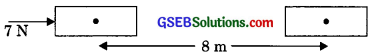Given, force applied, F = 7 N
Displacement, s = 8 m
Workdone, W = F x s
W = 7 x 8 = 56 J
W= 56 JPage 149

Question 1.
When do we say that work is done?
Conditions which are needed to be satisfied for work to be done are

• a force should act on an object.
• the object must be displaced.

Question 2.
Write an expression for the work done when a force is acting on an object in the direction of its displacement.
W = Fs
F = force
s = displacement

Question 3.
Define 1 J of work.
We know that, W = Fs
If F = 1 N and s = 1 m
W = 1 N x 1 m
1 J = 1 Nm
1 J is the amount of work done on an object when a force of 1 N displaces it by 1 m along the line of action
of the force.

Question 4.
A pair of bullocks exerts a force of 140 N on a plow. The field being plowed is 15 m long. How much work is done in plowing the length of the field?
Given, Force, F = 140 N
Displacement, s = 15 m
Workdone W = F x s
W = 140 x 15 = 2100 J

Page 152

Question 1.
What is the kinetic energy of an object?
The energy possessed by a body by the virtue of its motion is called kinetic energy.

Question 2.
Write an expression for the kinetic energy of an object.
Kinetic energy, EK = $$\frac {1}{2}$$ mυ2
m = mass of object
υ = velocity of object

Question 3.
The kinetic energy of an object of mass, m moving with a velocity of 5 m/s is 25 J. What will be its kinetic energy when its velocity is doubled? What will be its kinetic energy when its velocity is increased three times?
Given, kinetic energy, Ek = mυ2 = 25 J
velocity, υ = 5 m/s
using, Ek = $$\frac{1}{2}$$ mυ2
m = $$\frac{2 E_{K}}{v^{2}}$$
or, m = $$\frac{2 \times 25}{(5)^{2}}$$
∴ m = 2 Kg
Kinetic energy of object when its velocity is doubled. Velocity becomes, υ = 10 m/s
Ek = $$\frac{1}{2}$$mυ2 = $$\frac{1}{2}$$ x 2 x (10)2 = 100 J
Kinetic energy of object when its velocity is increased three times, i.e., velocity becomes, υ = 15 m/s
Ek = $$\frac{1}{2}$$mυ2= $$\frac{1}{2}$$ x 2 x (15)2 = 225JPage 156

Question 1.
What is power?
Power is defined as the rate of doing work or the rate of transfer of energy.
Power = work/time

Question 2.
Define 1 Watt of power.
We know that,
P = w/t
If w = 1J and t = 1s
P = $$\frac{1J}{1s}$$
∴ 1W = 1 J/s
1 watt is the power of an agent, which does work at a rate of 1 joule per second.

Question 3.
A lamp consumes 1000 J of electrical energy in 10 s. What is its power?
Given, Energy, E = 1000 J
Time, t = 10 s
Power, P = $$\frac{W}{t}$$ = $$\frac{E}{t}$$
or, P = $$\frac{1000 J}{10 s}$$
∴ P = 100 W

Question 4.
Define average power.
Average power is the ratio of the total work to the total time taken or the ratio of the total energy consumed to the total time taken.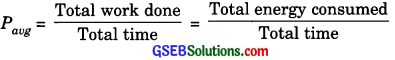In-Text Activities Solved

(Textbook Page 148)

Activity 11.4
Discussion and conclusion

• Work done by an external force is positive as the displacement takes place in the direction of the force.
• Work done by the gravitational force is negative as gravity is acting downward and displacement is upward.

(Textbook Page 149)

Activity 11.5
Discussion and conclusion

• Fossil fuels, solar energy, nuclear energy, wind energy, hydro energy, ocean energy, biomass, etc.
• Hydro-energy is the energy of flowing water and is due to water cycle in nature caused by solar energy, wind energy is due to the movement of air in the atmosphere due to non-uniform heating of the ground and water by solar energy.

(Textbook Page 150)

Activity 11.6
Discussion and conclusion
1. Here when ball drops, Its potential energy is converted into kinetic energy and finally resistive force offered by sand stops the ball.
2. Here the ball stops due to frictional force of sand.
Hence, mgh = F × x
F = friction force offered
x = depression in the sand bed
m = mass of the ball
h = height from which ball is dropped.
∴ x ∝ h
If ball is dropped from greater height, the dispersion will be greater.
Hence depression will be maximum (deepest) when the body is dropped from 1.5 m and minimum (shallowest) when body is dropped from 25 cm.(Textbook Page 150)

Activity 11.7discussion and conclusion
1. Here the potential energy of mass placed in the pan is converted into kinetic energy of the trolley which is finally transferred to the block.
2. If the mass in the pan increases, the amount of potential energy converted into kinetic energy increases. Hence displacement of the block is more.

(Textbook Page 152)

Activity 11.8
Discussion and conclusion
While stretching the rubber band, we are doing work done against restoring force. This work done is stored in the form of potential energy (elastic potential energy). When we release the band at one of the ends, its elastic potential energy is converted into kinetic energy.

(Textbook Page 152)

Activity 11.9
Discussion and conclusion

• Slinky tends to regain its original configuration.
• Work done in stretching is stored in the form Slinky of spring potential energy (Elastic potential energy).
• Yes, the slinky would also acquire elastic potential energy when it is compressed.(Textbook Page 152)

Activity 11.10
Discussion and conclusion

• Yes, it moves when placed on the ground.
• It acquires energy from the elastic potential energy of the spring.
• Yes, the energy acquired by the toy depends on the number of windings of the spring. When the spring of the toy is wound more, it stores more potential energy.
• In order to test it, give five windings to the spring of the toy and find the distance it covers on the ground. Now if ten windings are given to the spring of the toy. The toy covers a much large distance.

(Textbook Page 152)

Activity 11.11
Discussion and conclusion
As we are doing work against the gravitational field, when we are lifting the mass, this work done is stored in the form of gravitational potential energy.(Textbook Page 152)

Activity 11.12
Discussion and conclusion
When the arrow is released from the bow, it acquires its original configuration as the elastic potential energy of bow and stretched string is converted into kinetic energy of the arrow.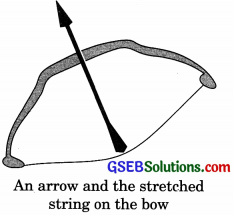(Textbook Page 154)

Activity 11.13
An arrow and the stretched
1. Conversion of electrical energy into sound energy: An electric bell, a stereo, a loudspeaker etc.
2. Conservation of heat energy into mechanical energy: In heat engines.
(a) Green plants produce food by photosynthesis.
(b) Plants get energy from the Sun.
(c) Air moves from one place to another place due to pressure difference. Pressure difference arises on account of non-uniform heating of the ground and seawater by solar radiation.
(d) Fossil fuels were formed millions of year ago, when plants and animal remains got buried under the earth and were subjected to high temperature and pressure conditions. Solar energy converts water into water vapor, increases the thermal energy of water.

Due to the buoyant force acting on water vapor its potential energy increases. The water vapor form clouds. When the cloud brings rain, the potential energy of the cloud is converted into kinetic energy of the raindrops.(Textbook Page 155)

Activity 11.15
Discussion and conclusion
1. For height h = 4 m,
Ep = mgh = 20 x 10 x 4 = 800 J
Ek = 0

2. For height h = 3 m,
Ep = mgh = 20 x 10 x 3 = 600J
Ek = $$\frac{1}{2}$$mυ2 = mg x = 20 x 10 x 1 = 200J

3. For height h = 2m,
Ep = mgh = 20 x 10 x 2 = 400J
Ek = $$\frac{1}{2}$$mυ2 = mg x = 20 x 10 x 2 = 400J

4. For height h = 1 m,
Ep = mgh = 20 x 10 x 1 = 200J
E k = $$\frac{1}{2}$$mυ2 = mg x = 20 x 10 x 3 = 600J

5. For height h = Just above the ground
Ep = mgh = 20 x 10 x 0 = 0 J
Ek = $$\frac{1}{2}$$mυ2 = mgx = 20 x 10 x 4 = 800J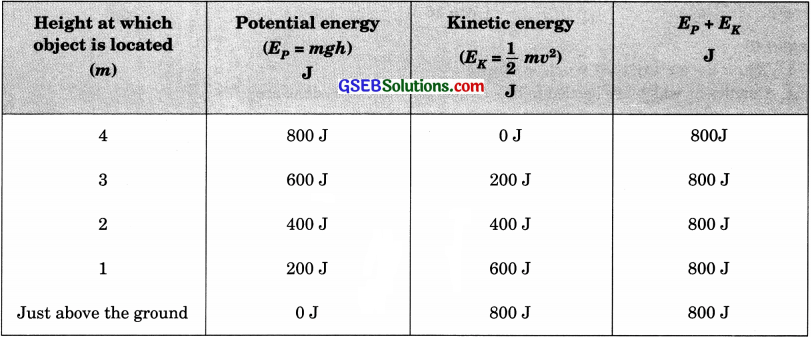(Textbook Page 155)

Activity 11.16
Discussion and conclusion
1. Here work done by both children is same.
Work done = Force x displacement
W = F x h = mgh = 80 mJ

2. Work was done per unit time by child A.
PA = $$\frac{W_{A}}{t_{A}}=\frac{80 \mathrm{m}}{15}$$
Work done per unit time by child B.
PB = $$\frac{W_{B}}{t_{B}}=\frac{80 \mathrm{m}}{20}$$
Here PA> PBThe power of child A is more than the power of child B.

### Gujarat Board Class 9 Science Work and Energy Textbook Questions and Answers

Question 1.
Look at the activities listed below. Reason out whether or not work is done in the light of your understanding of the term ‘work’.
(a) Suma is swimming in a pond.
(b) A donkey is carrying a load on its back.
(c) A windmill is lifting water from a well.
(d) A green plant is carrying out photosynthesis.
(e) An engine is pulling a train.
(f) Food grains are getting dried in the Suñ.
(g) A sailboat is moving due to wind energy.
(a) Suma is doing work as she moves by applying force with the muscles of her arms and lungs in water.
(b) A donkey is not doing any work as the force (weight) and displacement are perpendicular to each other.
(c) A windmill works against the gravitational force to lift water. Hence, work is done by the windmill in lifting water from the well.
(d) In this case, there is no displacement of leaves of the plant. Therefore, the work done is zero.
(e) An engine applies force to pull the train. This allows the train to move in the direction of force. Therefore, there is a displacement in the train in the same direction. Hence, work is done by the engine on the train.
(f) When the food grains are dried in the sun, solar radiation takes moisture out of food grains. Since there is no force and displacement is involved in the process, work done is zero.
(g) Wind energy applies a force on the sailboat to push it in the forward direction. Therefore, there is a displacement in the boat in the direction of the force. Hence, work is done by the wind on the boat.Question 2.
An object was thrown at a certain angle to the ground moves in a curved path and falls back to the ground. The initial and the final points of the path of the object lie on the same horizontal line. What is the work done by the force of gravity on the object?
Work done by the force of gravity on an object depends only on the vertical displacement. Vertical displacement is given by’the difference in the initial and final positions of the object, which is zero. Hence work done is zero. Here horizontal displacement is perpendicular to the direction of gravity.

Question 3.
A battery lights a bulb. Describe the energy changes involved in the process.
A battery converts chemical energy into electrical energy. This electrical energy is converted into light energy as the bulb is lighted up, i.e. the sequence of energy changes as follows: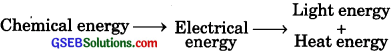Question 4.
The certain force acting on a 20 kg mass changes its velocity from 5 ms-1 to 2 ms-1. Calculate the work done by the force.
Given,
Mass of the body, m = 20 kg
Initial velocity, u = 5 rn/s
Final velocity, υ = 2 rn/s
Initial kinetic energy,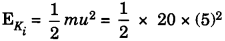= 250 J
Final kinetic energy,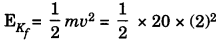= 40 J
Work done = change in kinetic energy
W= E -E
= 40 – 250 = -210 J
∴ W = – 210J
Negative sign is for retarding force.Question 5.
mass of 10 kg is at a point A on a table. It is moved to a point B. If the line joining A and B is horizontal, what is the work done on the object by the gravitational force? Explain your answer.
The work done by the gravitational force on the body is zero. This is due to the reason that force and displacement are perpendicular to each other.Question 6.
The potential energy of a freely falling object decreases progressively. Does this violate the law of conservation of energy? Why?
No, the process does not violate the law of conservation of energy. This is because when the body falls from a height, then its potential energy changes into kinetic progressively. A decrease in the potential energy is equal to an increase in the kinetic energy of the body. During the process, the total mechanical energy of the body remains conserved. Therefore, the law of conservation of energy is not violated.

Question 7.
What are the various energy transformations that occur when you are riding a bicycle?
While riding a bicycle, the muscular energy of the rider gets transformed into heat energy and kinetic energy of the bicycle. Heat energy heats the rider’s body. Kinetic energy provides velocity to the bicycle. The transformation can be shown as:
Muscular energy → Kinetic energy + heat energy
During this transformation, the total energy is conserved.Question 8.
Does the transfer of energy take place when you push a huge rock with all your might and fail to move it? Where is the energy you spend going?
When we push a huge rock and fail to move it, the energy spent in doing so is absorbed by the particle of the rock. The potential energy of the rock particles increases and deformation takes place. However, this deformation is not visible on account of the huge size of the rock.

Question 9.
A certain household has consumed 250 units of energy during a month. How much energy is this in joules?
1 unit of energy is equal to 1 kilowatt hour (kWh)
1 unit = 1 kWh
1 kWh = 3.6 x 106
Therefore, 250 units of energy = 250 x 3.6 x 106 = 9 x 108 J

Question 10.
An object of mass 40 kg is raised to a height of 5 m above the ground. What is its potential energy? If the object is allowed to fall, find its kinetic energy when it is half-way down.
Given,
mass of object, m = 40 kg
Vertical displacement, h = 5 m
The potential energy of mass, E = nigh
= 40 x 9.8 x 5 = 1960J
During free fall, its potential energy will be converted into kinetic energy. At half-height of potential energy will be converted into kinetic energy.
Ek = $$\frac {1960}{2}$$ = 980 JQuestion 11.
What is the work done by the force of gravity on a satellite moving round the earth? Justify your answer.
When a satellite rotates around the earth, the direction of force is perpendicular to the displacement for a short interval. Hence work done by gravitational force (gravity) is zero.

Question 12.
Can there be displacement of an object in the absence of any force acting on it? Think. Discuss this question with your friends and teacher.
Yes, for a body moving with the uniform velocity it is possible. The net force acting on it is zero. But, there is a displacement along with the motion of the object. Hence, there can be a displacement without a force.

Question 13.
A person holds a bundle of hay over his head for 30 minutes and gets tired. Has he done some work or not? Justify your answer.
When a person holds a bundle of hay on his head, then there is no displacement in the bundle of hay. Hence work done is zero but the person gets tired as he experiences muscular fatigue.Question 14.
An electric heater is rated 1500 W. How much energy does it use in 10 hours?
Given,
power of electric heater,
P = 1500W
Time for which heater is operated,
t = 10 h
Energy used by electric heater,
E = W x P x t
E = W = 1500W x 10 hr
= 15000 Whr = 15kWh = 15 units.

Question 15.
Illustrate the law of conservation of energy by discussing the energy changes which occur when we draw a pendulum bob to one side and allow it to oscillate. Why does the bob eventually come to rest? What happens to its energy eventually? Is it a violation of the law of conservation of energy?
When the pendulum bob is pulled, the energy supplied is stored in the form of potential energy on account of its higher position. When the pendulum is released so that it starts moving towards the right, then its potential energy changes into kinetic energy such that at the mean position, it has maximum kinetic energy and zero potential energy.

As the pendulum moves towards the extreme right, its kinetic energy changes into potential energy such that an extreme position, it has maximum potential energy and zero kinetic energy. When it moves from this extreme position to the mean position, its potential energy again changes to kinetic energy This illustrates the law of conservation of energy.

Eventually, the bob comes to rest, because during each oscillation a part of the energy possessed by it is transferred to air and in overcoming friction at the point of suspension. Thus, the energy of the pendulum is dissipated in the air. Here, the law of conservation of energy is not violated as energy is not destroyed but converted into another form.Question 16.
An object of mass, m is moving with a constant velocity, v. How much work should be done on the object in order to bring the object to rest?
Initial kinetic energy of body, $$E_{K_{i}}=\frac{1}{2} m v^{2}$$
Final kinetic energy of body, $$E_{K_{f}}$$ = 0
Work was done = change in kinetic energy
W = $$E_{K_{f}}-E_{K_{i}}=0-\frac{1}{2} m v^{2}$$
W = $$-\frac{1}{2} m v^{2}$$
The negative sign means that force is retarding in nature.

Question 17.
Calculate the work required to be done to stop a car of 1500 kg moving at a velocity of 60 km/h.
Given,
mass of car, m = 1500 kg
Initial velocity of car, u = 60 km/h
or, u = 60 x $$\frac {5}{18}$$ = $$\frac {50}{3}$$ m/s
Initial kinetic energy of care
$$E_{K_{i}}$$ = $$\frac {1}{2}$$mυ2
$$\frac {1}{2}$$ = 1500 x $$\left(\frac{50}{3}\right)^{2}$$
$$E_{K_{i}}$$ = 208333.3 J
Final kinetic energy of car,
$$E_{K_{f}}$$ = $$\frac {1}{2}$$mυ2 = 0
Work done = change in kinetic energy
W = E – E = 0 – 208333.3 J
w = – 2083333 JQuestion 18.
In each of the following a force, F is acting on an object of mass, m. The direction of displacement is from west to east shown by the longer arrow. Observe the diagrams carefully and state whether the work done by the force is negative, positive, or zero.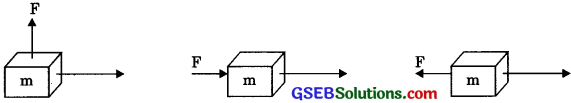• Work done is zero as force and displacement are perpendicular to each other.
• Work done is positive as force and displacement are in the same direction.
• Work done is negative as force and displacement are in opposite directions.

Question 19.
Soni says that the acceleration in an object could be zero even when several forces are acting on it. Do you agree with her? Why?
Yes, if the resultant force on the body is zero the acceleration will be zero.Hence, Soni is right.

Question 20.
Find the energy in kWh consumed in 10 hours by four devices of power 500 W each.